# Statistical Distributions for the Random Function (MathScript RT Module)

LabVIEW 2012 MathScript RT Module Help

Edition Date: June 2012

Part Number: 373123C-01

»View Product InfoDownload Help (Windows Only)

The following table describes the statistical distributions that the LabVIEW MathScript random function supports.

Distribution Equation Parameters
beta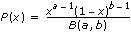for 0≤x≤1
a>0, b>0
binomial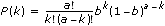for k=0, 1, ... , a
a is a positive integer; 0≤b≤1
chi-squared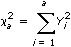where Yi have normal independent distributions with mean 0 and variance 1, and a is the degree of freedom.
a is a positive integer
exponential P(x) = aeax a>0
Fwhere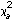and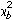have chi-square independent distributions with degrees of freedom a and b.
a and b are positive integers
gamma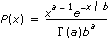a>0, b>0
geometric P(k) = a(1–a)k

for k=0, 1, 2, ...
0≤a≤1
hypergeometric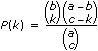where.
a, b, and c are positive integers, ba, ca
lognormal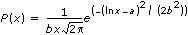a, b>0
negative binomial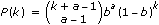for k=0, 1, 2, ...
a is a positive integer, 0≤b≤1
noncentral F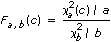where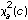andare two independently-distributed variables.
a and b are positive integers, c>0
noncentral T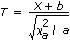where X andare two independently-distributed variables, X has a normal distribution, and a is the degree of freedom.
a is a positive integer, b
noncentral chi-square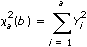where Yi have normal independent distributions with mean √(b/a) and variance 1, and a is the degree of freedom.
a is a positive integer, b>0
normal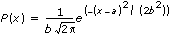a, b>0
Poisson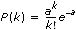for k=0, 1, 2, ...
a>0
Rayleigh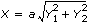where Yi have normal independent distributions with mean 0 and variance 1.
a>0
T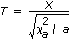where X andare two independently-distributed variables, X has a normal distribution, and a is the degree of freedom.
a is a positive integer
discrete uniform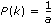for k = 1, 2, ..., a
a is a positive integer
continuous uniform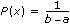for a<x<b
a<b
Weibull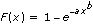a>0, b>0

Not Helpful